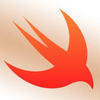## Welcome to the Treehouse Community

The Treehouse Community is a meeting place for developers, designers, and programmers of all backgrounds and skill levels to get support. Collaborate here on code errors or bugs that you need feedback on, or asking for an extra set of eyes on your latest project. Join thousands of Treehouse students and alumni in the community today. (Note: Only Treehouse students can comment or ask questions, but non-students are welcome to browse our conversations.)

### Looking to learn something new?

Treehouse offers a seven day free trial for new students. Get access to thousands of hours of content and a supportive community. Start your free trial today.# Need assistance with Swift generics

Since we don't want to interact with the array directly we need to add some code to let users know if the underlying data store contains elements. For this task add a computed property: isEmpty of type Bool that lets us know whether the queue is empty.

generics.swift
```struct Queue<Element> {

var array: [Element]

}
```

```public var isEmpty: Bool {
return array.isEmpty
}
```

It looks like you may encounter some bumps along the way, I recommend reading this article which seems that is what this challenge is based on:

https://www.raywenderlich.com/148141/swift-algorithm-club-swift-queue-data-structureI appreciate the assistance, but I have had this before and it does not complete the challenge

```struct Queue<Element> {

var array: [Element]

struct Queue<Element> {

var array: [Element]

public var isEmpty: Bool {
return array.isEmpty
}

}
```

Hi Jeff,

You need to initialize your variable stored property to an empty array before your computed property, isEmpty, will work:

```var array: [Element] = []
```

Now you should be able to solve the second challenge.Yes Sir - Here is how I completed it

```  mutating func dequeue() -> Element? {
if self.array.isEmpty == false {
return self.array.remove(at: 0)
} else {
return nil
}

}
```struct Queue<Element> {

```var array: [Element] = []
```

public var isEmpty: Bool { return array.isEmpty } var count : Int { return array.count
}

```mutating func enqueue(_ Value : Element) {

array.append(Value)
}
mutating func dequeue() -> Element? {
if self.array.isEmpty == false  {
return array.remove(at : 0)
}
else  {

return nil
}
```

}}

// HOPE ITS useful i have done this successfullyPLUS

struct Queue<Element> {

```var array: [Element] = []
```

public var isEmpty: Bool { return array.isEmpty } var count : Int { return array.count
}

```mutating func enqueue(_ Value : Element) {

array.append(Value)
}
mutating func dequeue() -> Element? {
if self.array.isEmpty == false  {
return array.remove(at : 0)
}
else  {

return nil
}
```

}}